Courses

# Linear Programming - Elements of Operation research, Business Mathematics and Statistics B Com Notes | EduRev

## B Com : Linear Programming - Elements of Operation research, Business Mathematics and Statistics B Com Notes | EduRev

The document Linear Programming - Elements of Operation research, Business Mathematics and Statistics B Com Notes | EduRev is a part of the B Com Course Business Mathematics and Statistics.
All you need of B Com at this link: B Com

Operations Research (OR) means a scientific approach to decision making, which seeks to determine how to optimally design and operate a system, usually under conditions requiring the allocation of scarce resources.

An OR study has the following major phases:

I. Define the problem and gather relevant data. ⇒

II. Formulate a mathematical model to represent the problem ⇒

III. Develop a procedure for deriving a solution to the problem ⇒

IV. Test the model and refine it as needed ⇒

V. Implement

Some of these phases will be illustrated the following example.

Prototype Example :

I. The GW firm manufactures two types of products W1 and W2 using two types of raw materials S1 and S2. The GW firm wishes to determine how many products of each type to produce, so as to achieve the highest possible profit.

II. The following data are available:

– 1 unit of W1 requires 2 kg of Sand 1 kg of S2.

– 1 unit of W2 requires 1 kg of S1 and 1 kg of S2.

– At present, GW has 100 kg of S1 and 80 kg of S2 in stock.

– Each unit of W1 gives a profit of \$3 and each unit of W2 gives a profit of \$2.

– Demand for Wequals 40 units and demand for Wis unlimited.

III. We define the decision variables: x1 - amount of product Wproduced, x2 amount of product W2 produced, Z - total profit from producing W1 and W2.

The mathematical model is as follows:

max Z = 3x1 + 2x2           [Maximize profit]

2x1 + x2 ≤ 100               [Constraint on raw material S1]

x1 + x2 ≤ 80                   [Constraint on raw material S2]

x1 ≤ 40                           [Demand for W1]

x1 ≥ 0                             [Sign restriction]

x2 ≥ 0                             [Sign restriction]

The problem is to choose values of x1 and x2, so as to maximize Z = 3x1 + 2xsubject to the restrictions. We want to derive a production plan that maximizes GW’s profit without violating the constraints on limited resources.

IV. We use an algorithm (the next part of the course will focus on the simplex method for solving linear programming problems). We obtain: x1 = 20, x2 = 60, Z = 180, so GW should produce 20 units of Wand 60 units of W2 with a resulting total profit of \$180.

THE LINEAR PROGRAMMING MODEL

The mathematical model for a general mathematical programming problem is as follows:

max(min)z = f(x1,...,xn)             [Objective function]

g1(x1,...,xn) ≤ (≥, =)b1                   [Constraint 1]

...

gm(x1,...,xn) ≤ (≥, =)bm            [Constraint m]

x1,...,xn are called decision variables (They should completely describe the decision to be made). A solution for which all the constraints are satisfied is called a feasible solution. A feasible solution with the largest (smallest) value of the objective function is called an optimal solution.

The function h(x1,...,xn) = a1x1+a2x2+ˇˇˇ+anxn of x1,x2,...,xn is a linear function.

A model in which all the functions f,g1,...,gm are linear is called a linear model or a linear programming problem. A linear programming model(LPM) is defined as follows:

max(min)z = c1x1 + c2x2 + ˇˇˇ + cnxn [Objective function]

a11x+ a12x2 + ˇˇˇ + a1nxn ≤ (≥, =)b1 [Constraint 1]

...

am1x1 + am2x2 + ˇˇˇ + amnxn ≤ (≥, =)bm [Constraint m]

x1 ≥ 0,...,xr ≥ 0, r ≤ n [Nonnegativity constraints]

The sign constraints xi ≥ 0 are special linear constraints (nonnegativity constraints).

EXAMPLES OF LINEAR PROGRAMMING

PROBLEMS

1. The Diet Problem. Person X requires that all the food she/he eats come from one of the four ”basic food groups”: butter, bread, orange juice and ham. She/he wants to prepare breakfast only from these foods. Each scoop of butter costs\$5, each slice (piece) of bread costs\$2, each bottle of orange juice

EXAMPLES OF LINEAR PROGRAMMING PROBLEMS

costs \$3, and each piece (slice) of ham costs \$8. Each day X must ingest at least 500 calories, 6 oz of sugar, 10 oz of carbohydrates, and 8 oz of fat. The nutritional content per unit of each food is shown in the following table: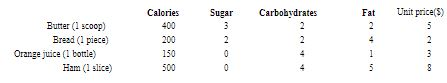Formulate a linear programming model that satisfies the daily nutritional requirements of person X at minimum cost.

Decision variables:

x1 - amount(number of scoops) of butter,

x2 - amount(number of pieces) of bread,

x3 - number of bottles of orange juice,

x4 - amount (number of slices) of ham.

Linear Programming Model:

min Z = 5x1 + 2x2 + 3x3 + 8x4                      [Minimize total cost]

400x1 + 200x+ 150x3 + 500x4 ≥ 500      [Calorie constraint]

3x1 + 2x2 ≥ 6                                             [Sugar constraint]

2x1 + 2x2 + 4x3 + 4x4 ≥ 10                        [Carbohydrates constraint]

2x1 + 4x2 + x3 + 5x≥ 8                             [Fat constraint]

xi ≥ 0, i = 1,..., 4                                         [Sign constraint]

The optimal solution to this problem (solved by computer) is x1 = 0, x2 = 3, x3 = 1, x4 = 0, Z = 9. Thus, the minimum-cost diet incurs a cost of \$9 and consists of 3 pieces of bread and one bottle of orange juice.

2. Production Process Model ( W.L. Winston Operations Research: Applications and Algorithms, Example 3.11, pp.87-90) Rylon Corporation manufactures four types of perfumes: Brute, Chanelle, Super Brute and Super Chanelle.

The production process is given in the Figure below.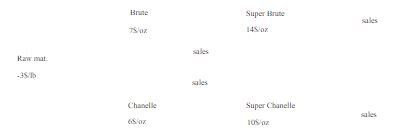• Processing 1 lb of raw material requires 1 hour of working time and gives 3 oz of Brute and 4 oz of Chanelle.

• 1 oz of Brute requires an additional 3 hours of processing to yield 1 oz of Super Brute.

• 1 oz of Chanelle requires an additional 2 hours of processing to yield 1 oz of Super Chanelle

•  Rylon has 6000 hours of time available and can purchase up to 4000 lb of raw material.

R.C must determine how much material to purchase and how much of each type of perfume should be produced and wants to maximize profit(=revenues from perfume sales - processing costs).

Decision variables:

• x1 - number of ounces of Brute sold,

• x2 - number of ounces of Super Brute sold,

• x3 - number of ounces of Chanelle sold,

• x4 - number of ounces of Super Chanelle sold,

• x5 - number of pounds(lb) of raw material purchased.

Linear Programming Model:

max z = 7x1 + 14x2 + 6x3 + 10x4 − 3x5         [Maximize profit]

x1 + x2 − 3x5 = 0                                             [Manufacturing Brute and Super Brute]

x3 + x4 − 4x= 0                                             [Manufacturing Chanelle and Super Chanelle]

x5 ≤ 4000                                                         [Limit on raw materials]

3x2 + 2x4 + x5 ≤ 6000                                      [Limit on working time]

xi ≥ 0, i = 1,..., 5                                                [Sign restrictions]

After solving the model by computer we obtain the following optimal solution:

Z=172 666.667\$ (profit), x1 = 11333.333 oz of Brute, x2 = 666.667 oz of Super Brute, x3 = 16000 oz of Chanelle, x4 = 0 oz of Super Chanelle and x5 = 4000lb of raw material.

3. An Inventory Model - Multiperiod Decision problems. A factory must produce a certain product over the next four quarters. The demand for each quarter is known. The factory wants to minimize total costs and has to meet all demands on time. The appropriate data are given in the following table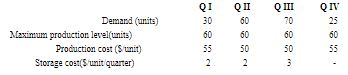Decision variables:

• x- number of units produced during quarter i, i = 1,... 4.

• m- number of units of the product in store (on the inventory) at the end of quarter i, i = 1,... 4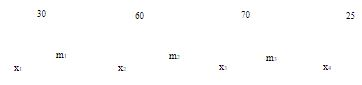Linear Programming Model:

min z = 55x+ 50x2 + 50x3 + 55x4 + 2m1 + 2m2 + 3m3      [Minimum total cost]

x− m1 = 30                                                                        [Balance for I]

m1 + x2 − m= 60                                                                [Balance for II]

m2 + x3 − m3 = 70                                                              [Balance for III ]

m3 + x= 25                                                                        [Balance for IV]

xi ≤ 60, i = 1,... 4                                                                  [Production capacity]

x≥ 0, m≥ 0, i = 1,..., 4                                                       [Sign restrictions]

The optimal solution to this problem is Z = 9615: x1 = 40, x2 = 60, x3 = 60, x4 = 25, m1 = 10, m2 = 10, m3 = 0.

4. Multiperiod Financial Model. (W.L. Winston Operations Research: Appli cations and Algorithms, Example 3.13, pp.95-97) Finco Invest. Corp. must determine an investment strategy for the next three years. At present (time 0), \$100000 is available for investment. Investments A,B,C,D and E are available. The cash flow associated with investing \$1 in each investment is given in the table below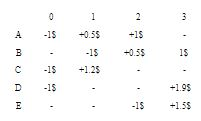For example, \$1 invested in B requires \$1 in cash at time 1 and returns \$0.5 at time 2 and \$1 at time 3. To ensure that the company’s portfolio is diversified, Finco requires that at most \$75000 be placed in any single investment. In addition, Finco can earn interest at 8% per year by keeping uninvested cash in a bank. Returns from investments may be immediately reinvested. Formulate a linear programming problem whose solution will maximize the amount of cash in hand at time 3. Finco must decide how much money should be placed in each investment(including the bank).

Decision variables:

• xA,xB,xC ,xD,xE - dollars invested in investment A,B,C,D, and E respectively,

• y0,y1,y2 - dollars invested in the bank at time t (t=0,1,2).

The linear programming model:

max z = xB + 1.9xD + 1.5xE + 1.08y2           [Maximize the amount of cash in hand at time 3]

xA + xC + xD + y0 = 100000                          [Balance at time 0]

0.5x+ 1.2xC + 1.08y0 − xB − y1 = 0           [Balance at time 1]

xA + 0.5xB + 1.08y1 − xE − y2 = 0                 [Balance at time 2]

xA,xB,xC ,xD,xE ≤ 75000                               [Limit on dollars invested in investments]

xA,...,xE,y0,y1,y2 ≥ 0                                     [Nonnegativity constraints]

The optimal solution is Z = 218500, xA = 60000, xB = 30000, xD = 40000 , xE = 75000, xC = y0 = y1 = y2 = 0. Finco should not invest in C nor in the bank.

Offer running on EduRev: Apply code STAYHOME200 to get INR 200 off on our premium plan EduRev Infinity!

122 videos|142 docs

,

,

,

,

,

,

,

,

,

,

,

,

,

,

,

,

,

,

,

,

,

,

,

,

;